# Simplex Algorithm – Tabular Method

Simplex Algorithm is a well-known optimization technique in Linear Programming.
The general form of an LPP (Linear Programming Problem) isExample: Let’s consider the following maximization problem.Initial construction steps :

• Build your matrix A. A will contain the coefficients of the constraints.
• Matrix b will contain the amount of resources.
• And matrix c will contain the coefficients of objective function or cost.

For the above problem –
Matrix A – At Iteration 0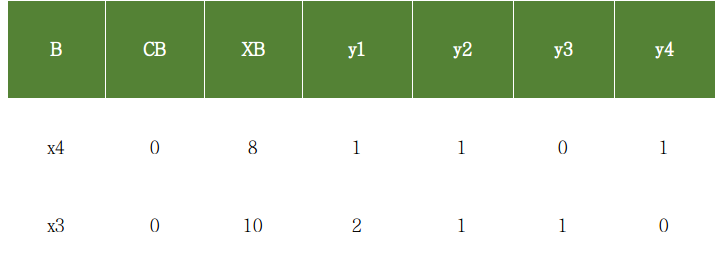At Iteration 0

Explanation of table-
B : Basis and contains the basic variables. Simplex algorithm starts with those variables which form an indentity matrix. In the above eg x4 and x3 forms a 2×2 identity matrix.
CB : Its the coefficients of the basic variables in the objective function. The objective functions doesn’t contain x4 and x3, so these are 0.
XB : The number of resources or we can say the RHS of the constraints.
yi : The complete Matrix A.

Simplex Algorithm
2. Calculate the relative profits.

For MAX problem-
If all the relative profits are less than or equal to 0, then the current basis is
the optimal one. STOP.
Else contniue to 3.

For MIN problem
If all the relative profits are greater than or equal to 0, then the current basis is
the optimal one. STOP.
Else contniue to 3.

3. Find the column corresponding to max relative profit. Say column k has the max
Rel. profit. So xk will enter the basis.

4. Perform a min ratio test to determine which variable will leave the basis.Index of the min element i.e 'r' will determine the leaving variable.
The basic variable at index r, will leave the basis.
NOTE: Min ratio test is always performed on positive elements.

5. It's evident that the entered variable will not form an identity matrix, so
we will have to perform row operations to make it identity again.
Find the pivot element. The element at index (r, k) will be the pivot element and
row r will be the pivot row.

6. Divide the rth row by pivot to make it 1. And subtract c*(rth row) from other
rows to make them 0, where c is the coefficient required to make that row 0.


Table at Iteration 1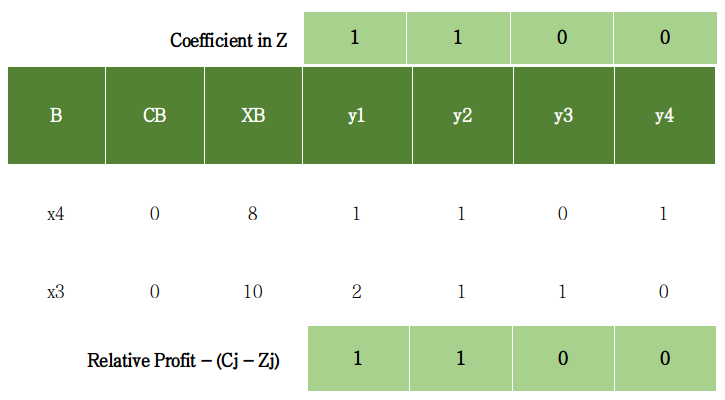Table at iteration 1

Calculation of relative profits – (Cj – Zj), where Cj is the coefficient in Z and Zj is yi*CB
C1 – Z1 = 1 – (1*0 + 2*0)
C2 – Z2 = 1 – (1*0 + 1*0)
C3 – Z3 = 0 – (0*0 + 1*0)
C4 – Z4 = 0 – (1*0 + 0*0)

So Relative profits are- 1, 1, 0, 0 (As shown in the table)
Clearly not all the relative profits are less or equal to 0. So will perform the next iteration.
Determination of entering variable and leaving variable.
Max relative profit 1 at index 1. So x1 will enter basis.Min of (8, 5) is 5 which is at index 2. So x3 will leave the basis.

Since x1 entered perform required row operations to make idenity matrix.

Pivot index = [2, 4]
Pivot element = 2

Divide the 2nd row by pivot element i.e 2 to make it 1.
And subtract 1*R2 from R1 to make it 0
See next table.

Table At Iteration 2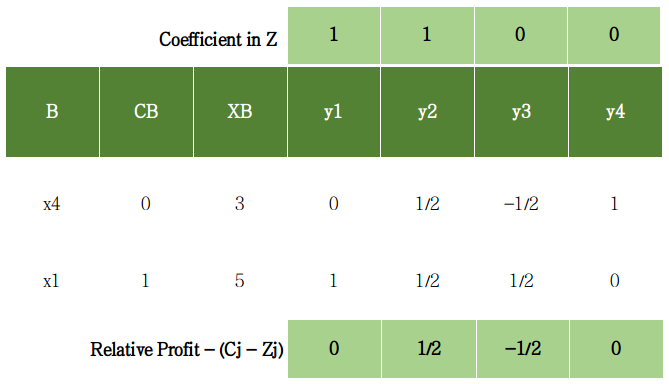Table at iteration 2

Relative profits = 0, 1/2, -1/2, 0
Pivot index = [1, 5]
Pivot element = 1/2
Perform necessary row operations.
See next table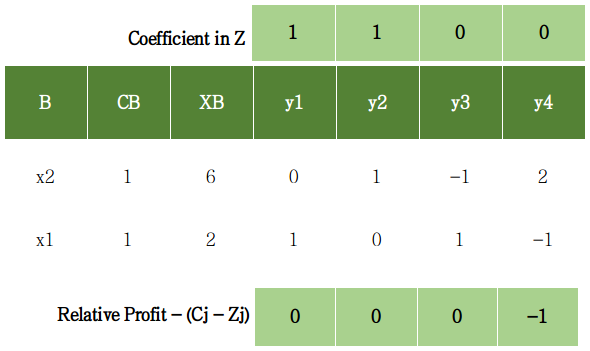Table At iteration 3

Relative profits = 0, 0, 0, -1
Since all relative profits are less than or equal to 0. So optimality is reached.
This will be the final simplex table and the optimal one.
Value of Z at optimality = 6*1 + 2*1 = 8

Following cases can occur while performing this algorithm.

• Case 1 – Unbounded Solution
If the column corresponding to the max relative profit contains only non-positive real numbers then we won’t be able to perform the min ratio test. Therefore it is reported as unbounded solution.
• Case 2 – Alternate Solution
If at any iteration any one of the non-basic variable’s relative profit comes out to be 0, then it contains alternate solutions. Many optimal solutions will exist.

Example 2
The above example was an equality case where we were able to find the initial basis. Now we will perform simplex on an example where there is no identity forming.Convert the above problem into standard form i.ewhere x3, x4 and x5 are slack variables. These will form identity and hence the initial basis.
Table at Iteration 0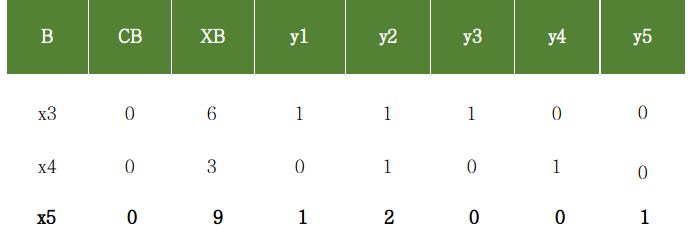Table at iteration 0

Now continuing as the previous example.
Table at iteration 1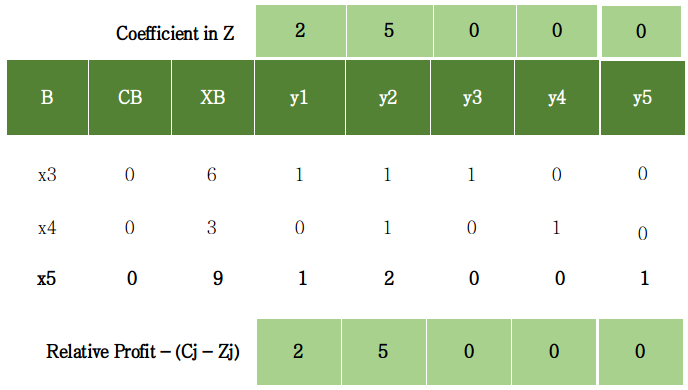Table at iteration 1

Relative profits = 2, 5, 0, 0, 0
Pivot Index = [2, 5]
Pivot element = 1

Table at Iteration 2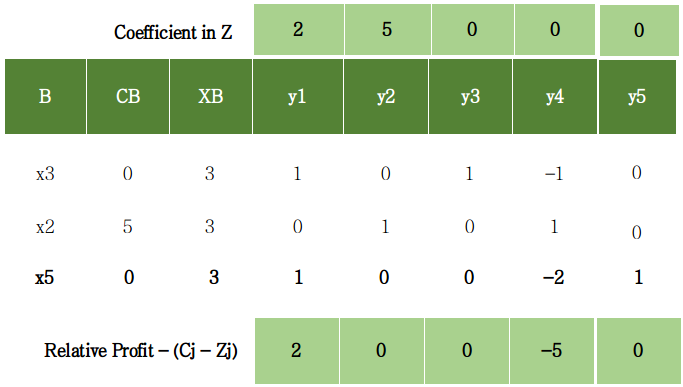Table at iteration 2

Relative Profits = 2, 0, 0, -5, 0
Pivot Index = [1, 4]
Pivot Element = 1

Table at iteration 3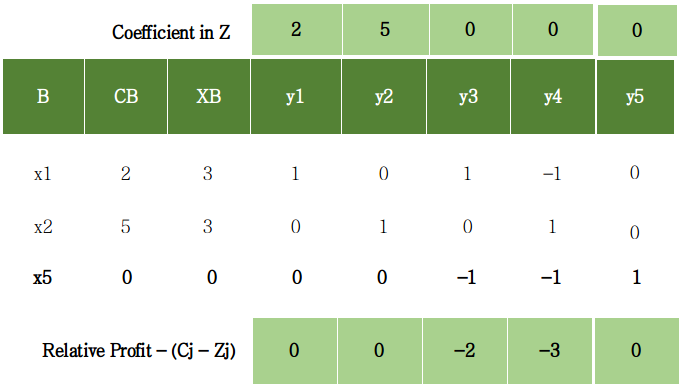Table at iteration 3

Relative profits = 0, 0, 0, -2, -3, 0
Since all relative profits are less than equal to 0. Optimality is reached.
This is the final simplex table and the optimal one.
Value of Z at optimality = 3*2 + 3*5 + 0*0 = 21

Code Implementation of Simplex Algorithm

 import numpy as np   from fractions import Fraction # so that numbers are not displayed in decimal.     print("\n                 ****SiMplex Algorithm ****\n\n")     # inputs      # A will contain the coefficients of the constraints  A = np.array([[1, 1, 0, 1], [2, 1, 1, 0]])  # b will contain the amount of resources   b = np.array([8, 10])             # c will contain coefficients of objective function Z        c = np.array([1, 1, 0, 0])                  # B will contain the basic variables that make identity matrix  cb = np.array(c)  B = np.array([, ])             # cb contains their corresponding coefficients in Z     cb = np.vstack((cb, c))          xb = np.transpose([b])                   # combine matrices B and cb  table = np.hstack((B, cb))               table = np.hstack((table, xb))           # combine matrices B, cb and xb  # finally combine matrix A to form the complete simplex table  table = np.hstack((table, A))           # change the type of table to float  table = np.array(table, dtype ='float')   # inputs end     # if min problem, make this var 1  MIN = 0    print("Table at itr = 0")  print("B \tCB \tXB \ty1 \ty2 \ty3 \ty4")  for row in table:      for el in row:                  # limit the denominator under 100          print(Fraction(str(el)).limit_denominator(100), end ='\t')       print()  print()  print("Simplex Working....")     # when optimality reached it will be made 1  reached = 0      itr = 1 unbounded = 0 alternate = 0    while reached == 0:         print("Iteration: ", end =' ')      print(itr)      print("B \tCB \tXB \ty1 \ty2 \ty3 \ty4")      for row in table:          for el in row:              print(Fraction(str(el)).limit_denominator(100), end ='\t')          print()         # calculate Relative profits-> cj - zj for non-basics      i = 0     rel_prof = []      while i<len(A):          rel_prof.append(c[i] - np.sum(table[:, 1]*table[:, 3 + i]))          i = i + 1        print("rel profit: ", end =" ")      for profit in rel_prof:          print(Fraction(str(profit)).limit_denominator(100), end =", ")      print()      i = 0            b_var = table[:, 0]      # checking for alternate solution      while i<len(A):          j = 0         present = 0         while j<len(b_var):              if int(b_var[j]) == i:                  present = 1                 break;              j+= 1         if present == 0:              if rel_prof[i] == 0:                  alternate = 1                 print("Case of Alternate found")                  # print(i, end =" ")          i+= 1     print()      flag = 0     for profit in rel_prof:          if profit>0:              flag = 1             break         # if all relative profits <= 0      if flag == 0:          print("All profits are <= 0, optimality reached")          reached = 1         break        # kth var will enter the basis      k = rel_prof.index(max(rel_prof))      min = 99999     i = 0;      r = -1     # min ratio test (only positive values)      while i<len(table):          if (table[:, 2][i]>0 and table[:, 3 + k][i]>0):               val = table[:, 2][i]/table[:, 3 + k][i]              if val<min:                  min = val                  r = i     # leaving variable          i+= 1            # if no min ratio test was performed      if r ==-1:          unbounded = 1         print("Case of Unbounded")          break        print("pivot element index:", end =' ')      print(np.array([r, 3 + k]))         pivot = table[r][3 + k]      print("pivot element: ", end =" ")      print(Fraction(pivot).limit_denominator(100))                     # perform row operations      # divide the pivot row with the pivot element      table[r, 2:len(table)] = table[              r, 2:len(table)] / pivot                     # do row operation on other rows      i = 0     while i<len(table):          if i != r:              table[i, 2:len(table)] = table[i,                   2:len(table)] - table[i][3 + k] *                   table[r, 2:len(table)]          i += 1               # assign the new basic variable      table[r] = k      table[r] = c[r]             print()      print()      itr+= 1           print()     print("***************************************************************")  if unbounded == 1:      print("UNBOUNDED LPP")      exit()  if alternate == 1:      print("ALTERNATE Solution")     print("optimal table:")  print("B \tCB \tXB \ty1 \ty2 \ty3 \ty4")  for row in table:      for el in row:          print(Fraction(str(el)).limit_denominator(100), end ='\t')      print()  print()  print("value of Z at optimality: ", end =" ")     basis = []  i = 0 sum = 0 while i<len(table):      sum += c[int(table[i])]*table[i]      temp = "x"+str(int(table[i])+1)      basis.append(temp)      i+= 1 # if MIN problem make z negative  if MIN == 1:      print(-Fraction(str(sum)).limit_denominator(100))  else:      print(Fraction(str(sum)).limit_denominator(100))  print("Final Basis: ", end =" ")  print(basis)     print("Simplex Finished...")  print()

For the above just plug in the required values and you will get a detailed step by step solution of your LPP by simplex algorithm.

My Personal Notes arrow_drop_upCheck out this Author's contributed articles.

If you like GeeksforGeeks and would like to contribute, you can also write an article using contribute.geeksforgeeks.org or mail your article to contribute@geeksforgeeks.org. See your article appearing on the GeeksforGeeks main page and help other Geeks.

Please Improve this article if you find anything incorrect by clicking on the "Improve Article" button below.

Article Tags :

1

Please write to us at contribute@geeksforgeeks.org to report any issue with the above content.